Question

A 119-mL bubble of hot gases at 217 ∘C and 1.76 atm escapes from an active volcano.

What is the temperature, in ∘C, of the gas in the bubble outside the volcano if the new volume of the bubble is 149 mL and the pressure is 0.739 atm ?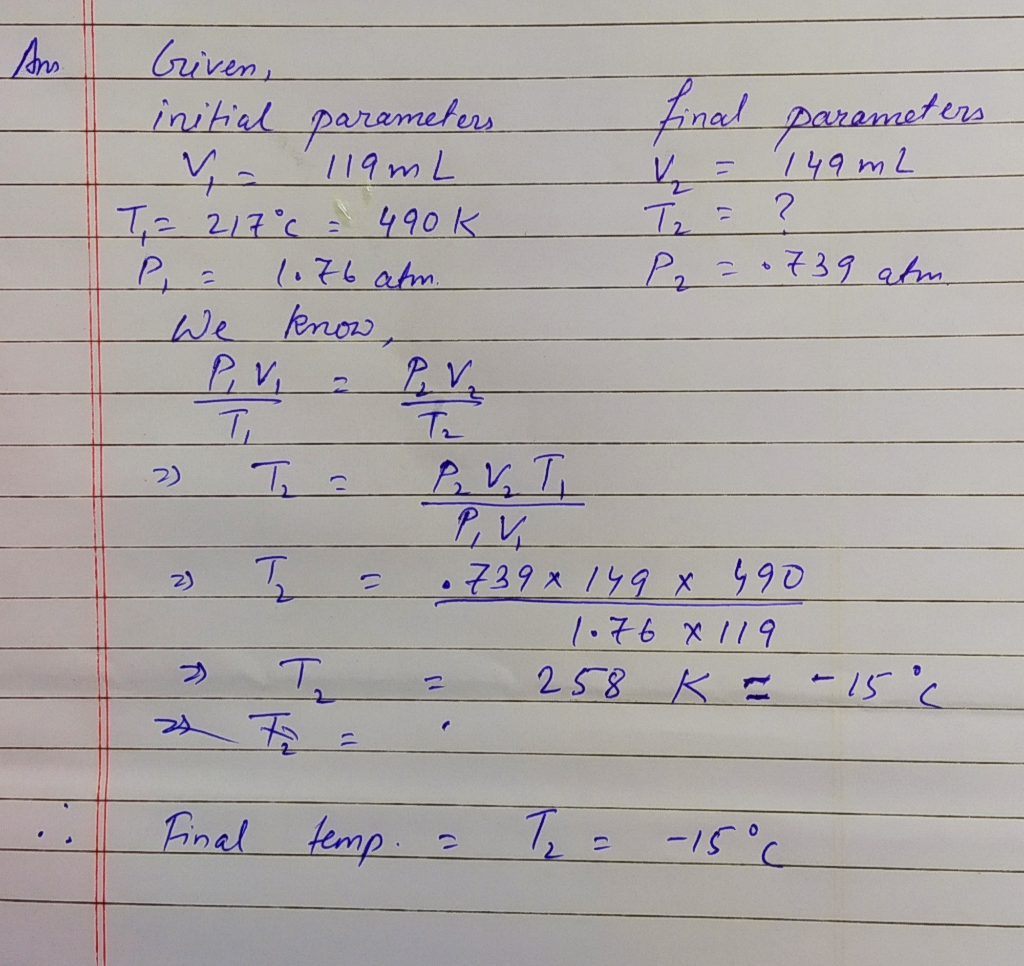#### Earn Coins

Coins can be redeemed for fabulous gifts.

Similar Homework Help Questions
• ### A 121.0-ml bubble of hot gases at 241 °C and 1.80 atm escapes from an active...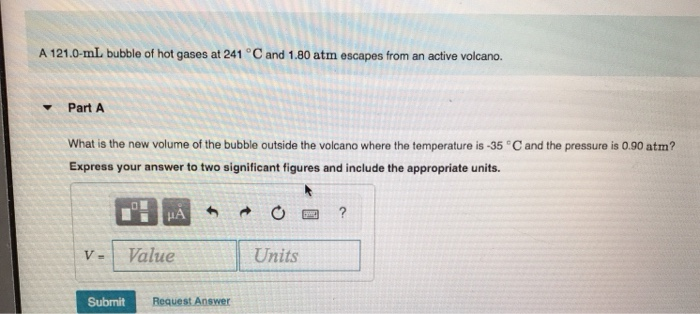A 121.0-ml bubble of hot gases at 241 °C and 1.80 atm escapes from an active volcano. Part A What is the new volume of the bubble outside the volcano where the temperature is -35°C and the pressure is 0.90 atm? Express your answer to two significant figures and include the appropriate units. HA O ? Value Units Submit Request Answer

• ### A 149.0-mL bubble of hot gases at 225 °Cand 1.60 atm escapes from an active volcano...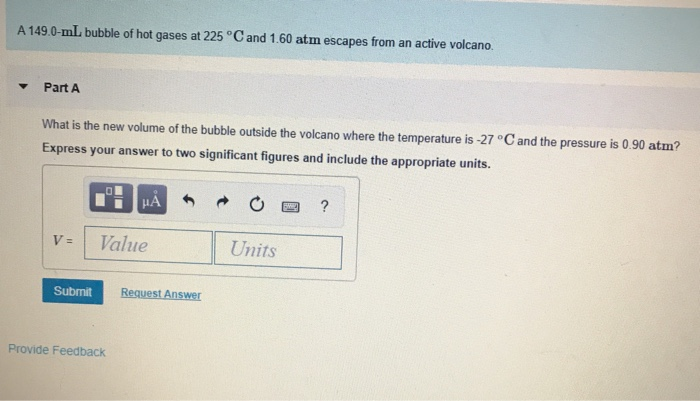A 149.0-mL bubble of hot gases at 225 °Cand 1.60 atm escapes from an active volcano Part A What is the new volume of the bubble outside the volcano where the temperature is -27 C and the pressure is 0.90 atm? Express your answer to two significant figures and include the appropriate units. ? Value Units V= Request Answer Submit Provide Feedback

• ### A 127 mL bubble of hot gases at 213 ∘C and 1.78 atm is emitted from...

A 127 mL bubble of hot gases at 213 ∘C and 1.78 atm is emitted from an active volcano. Part A What is the final temperature, in degrees Celsius, of the gas in the bubble outside the volcano if the final volume of the bubble is 136 mL and the pressure is 0.770 atm , if the amount of gas does not change? Express your answer using two significant figures.

• ### Review I Constants I Periodic Table A 128 mL bubble of hot gases at 213 °...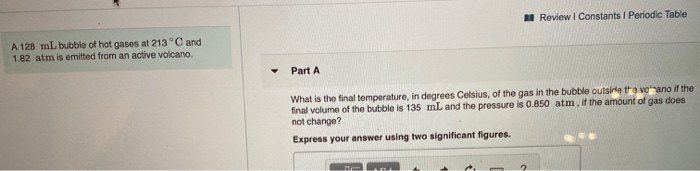Review I Constants I Periodic Table A 128 mL bubble of hot gases at 213 ° C and 1.82 atm is emitted from an active volcano. Part A What is the final temperature, in degrees Celsius, of the gas in the bubble outsine tha voano if the final volume of the bubble is 135 mL and the pressure is 0.850 atm, if the amount of gas does not change? Express your answer using two significant figures.

• ### Problem 8.45 19 of 20 Review Constants Periodic Table Part A A 126 mL bubble of...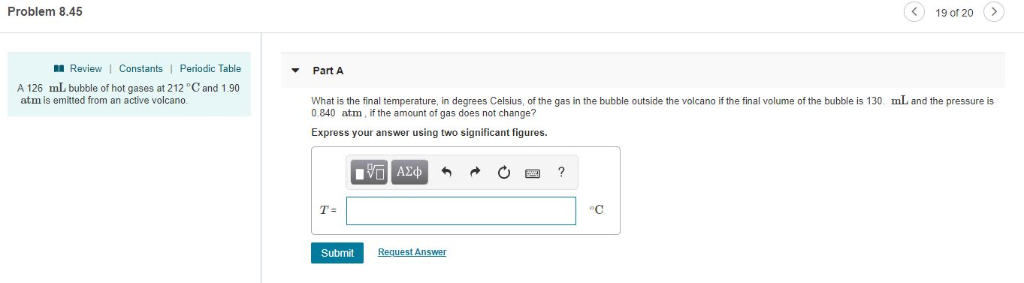Problem 8.45 19 of 20 Review Constants Periodic Table Part A A 126 mL bubble of hot gases at 212 °C and 1.90 atm is emitted from an active volcano. mL and the pressure is f the gas in the bubble outside the volcano if the final volume of the bubble is 130 0840 atm, if the amount of gas does not change? Express your answer using two significant figures. ν ΑΣφ T- Request Answer Submit

• ### Part 1) Part 2) Part B A sample of argon gas has a volume of 735...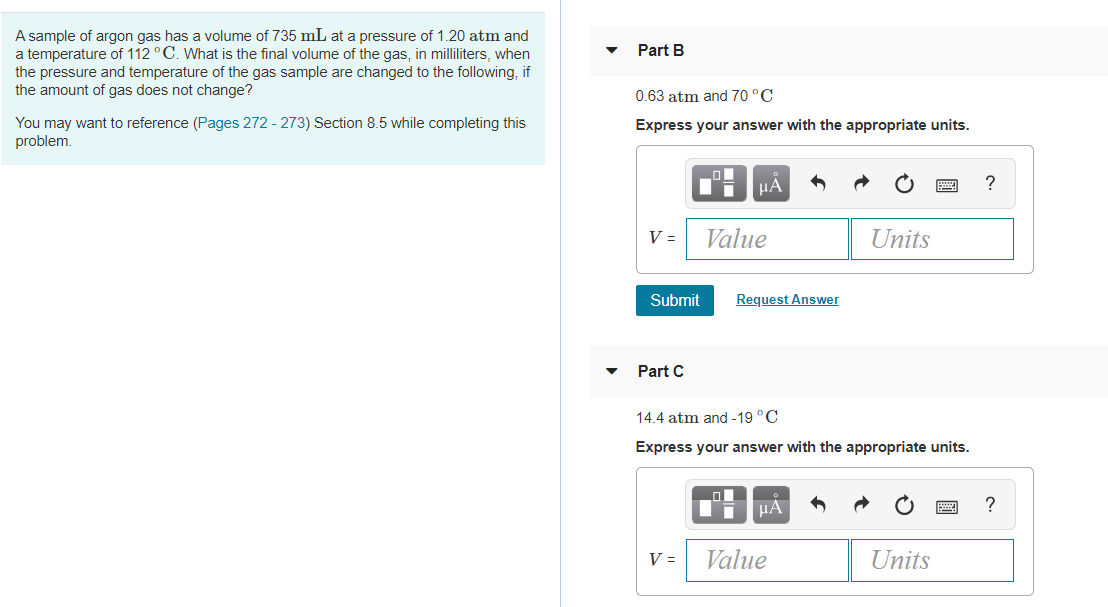Part 1) Part 2) Part B A sample of argon gas has a volume of 735 mL at a pressure of 1.20 atm and a temperature of 112 °C. What is the final volume of the gas, in milliliters, when the pressure and temperature of the gas sample are changed to the following, if the amount of gas does not change? 0.63 atm and 70°C Express your answer with the appropriate units. You may want to reference (Pages 272-273) Section...

• ### A diver exhales a bubble with a volume of 250 mL at a pressure of 2.4 atm and a temperature of 15 °C

A diver exhales a bubble with a volume of 250 mL at a pressure of 2.4 atm and a temperature of 15 °C. How many gas particles are in this bubble?

• ### Consider the apparatus shown in the drawing O2 2.0 L 1.0 atm 25°C 3.0 L 2.0 atm 25℃ Part A When the valve between the two containers is opened and the gases allowed to mix, how does the volume occupi...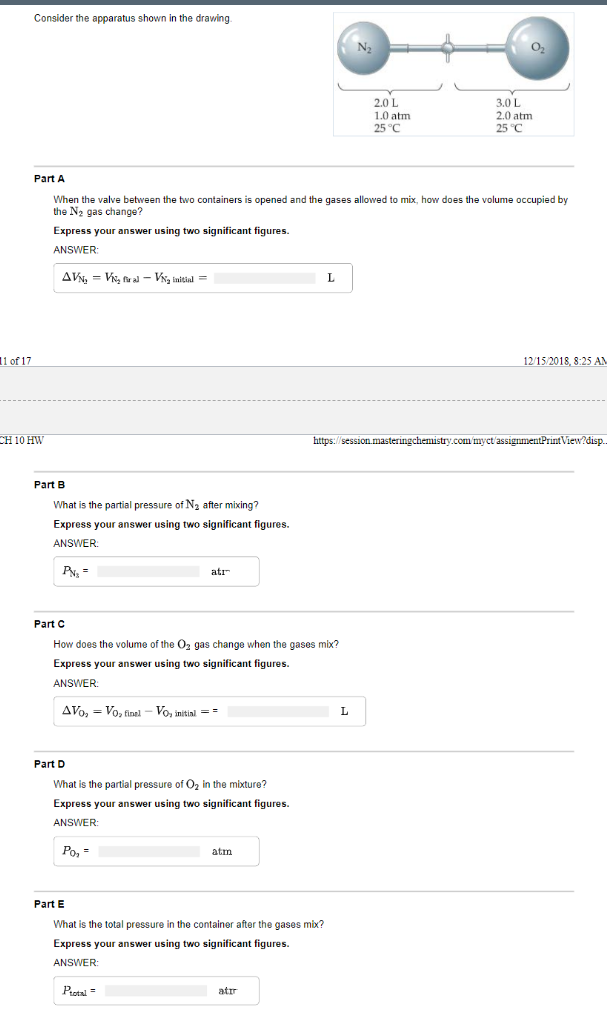Consider the apparatus shown in the drawing O2 2.0 L 1.0 atm 25°C 3.0 L 2.0 atm 25℃ Part A When the valve between the two containers is opened and the gases allowed to mix, how does the volume occupied by the N2 gas change? Express your answer using two significant figures. ANSWER of 17 215/2018, 8:25 A com myct assignmentPrint View?disp. CH 10 HW Part B What is the partial pressure of N2 after mixing? Expre ANSWER ss your...

• ### A sample of an ideal gas at 1.00 atm and a volume of 1.76 L was placed in a weighted balloon and dropped into the ocean.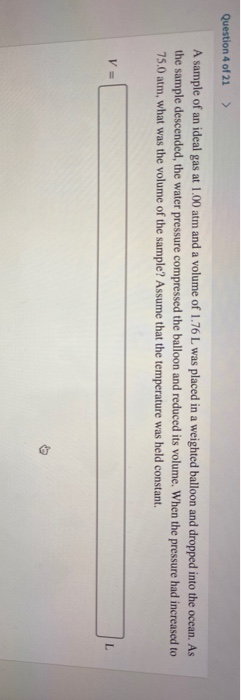Question 4 of 21 A sample of an ideal gas at 1.00 atm and a volume of 1.76 L was placed in a weighted balloon and dropped into the ocean. As the sample descended, the water pressure compressed the balloon and reduced its volume. When the pressure had increased to 75.0 atm, what was the volume of the sample? Assume that the temperature was held constant.Questions of 21 A hot air balloon is filled with 1.31 x 10 L of an ideal...

• ### A diver exhales a bubble with a volume of 25 mL at a pressure of 2.4 atm and a temperature of 15 degrees C

A diver exhales a bubble with a volume of 25 mL at a pressure of 2.4 atm and a temperature of 15 degrees C. how many gas particles are in this bubble?